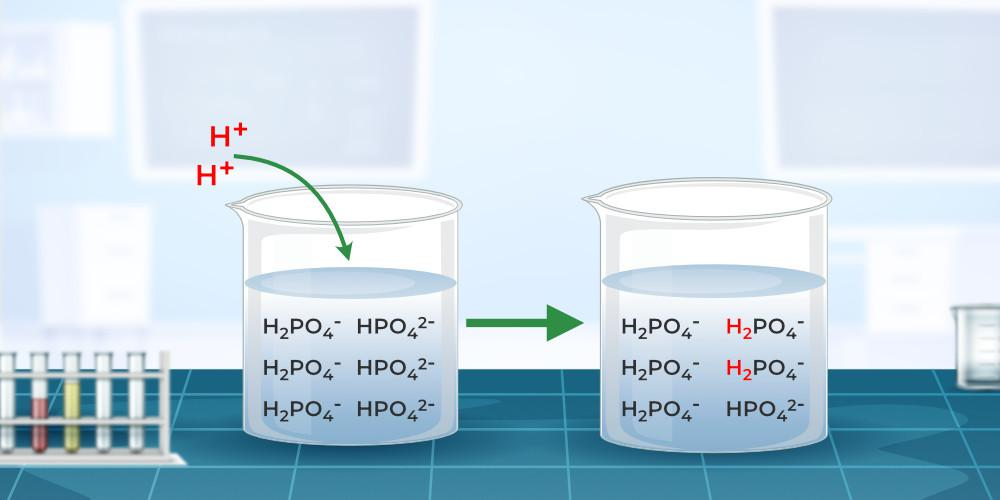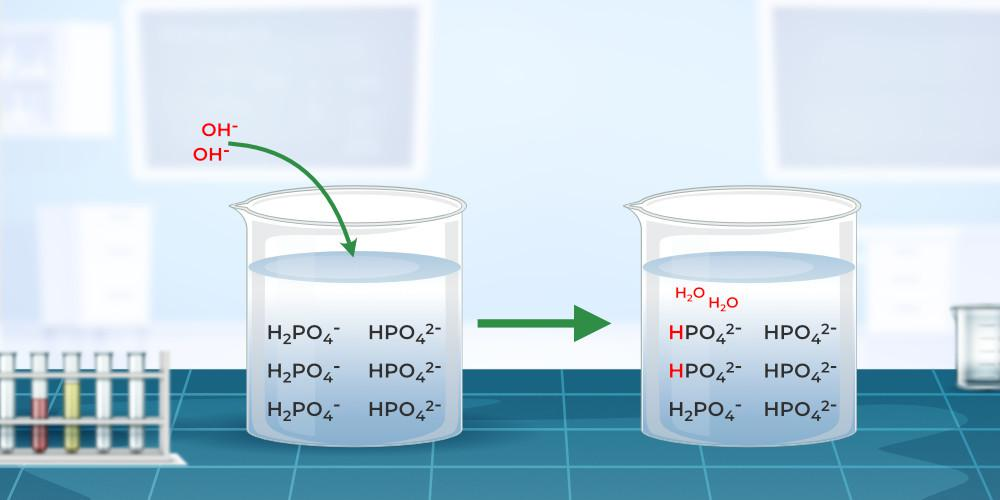GeeksforGeeks App
Open AppBrowser
Continue

## Related Articles

• CBSE Class 11 Chemistry Notes

Buffer Solution is a special aqueous solution that resists the change in its pH when some quantity of acid and Base is added. Many fluids, such as blood, have specific pH values of 7.14, and variations in these values indicate that the body is malfunctioning.  The change in pH of Buffer Solutions on adding a small quantity of acid or bases is very minimal and hence they are used to make solutions that resist the change in pH.

## Buffer Solution Definition

A buffer solution is a solution that resists changes in hydrogen ion concentration when a modest amount of acid or base is added. In other words, such solutions are known to have reverse acidity and reverse basicity and to keep a reasonably steady pH value. A good example of a natural buffer solution is human blood. Despite eating a wide array of meals, our blood maintains a pH of roughly 7.35.

Buffer Solution is a water-based solvent-based solution made up of a weak acid and its conjugate base, or a weak base and its conjugate acid. They are resistant to changes in pH caused by dilution or the addition of relatively small amounts of acid or alkali. When a small amount of strong acid or strong base is added, the pH of the buffer solution changes very little. As a result, they’re used to maintaining a steady pH. When a tiny amount of strong acid or base is given to it, its pH varies very little, and it is thus used to keep a solution’s pH stable.

A buffer solution is one that can maintain its hydrogen ion concentration (pH) with just slight dilution or the addition of a small amount of acid or base. Fermentation, food preservation, medicine administration, electroplating, printing, enzyme activity, and blood oxygen-carrying capability all require particular hydrogen ion concentrations (pH) in buffer solutions. Buffer solutions are made up of a weak acid and its conjugate base or a weak base and its conjugate acid that can maintain pH.

## Types of Buffer Solutions

Acidic and basic buffers are the two types of buffer solutions that are extensively classified. These are discussed in greater depth down below.

### Acidic Buffers

Acidic buffer solutions are made up of equimolar amounts of a weak acid and its salt, as well as a strong base. These solutions are used to keep the environment acidic. An acid buffer is made by combining a weak acid and its salt with a strong base to create an acidic pH.

The pH of an aqueous solution containing an equal amount of acetic acid and sodium acetate is 4.74. Furthermore, the pH of these liquids is less than seven. These solutions are made up of a weak acid and its salt.### Basic or Alkaline Buffers

A weak base and its salt are equimolar with a strong acid in a basic buffer solution. These buffer solutions are employed to keep basic conditions. To generate a basic buffer with a basic pH, a weak base and its salt are combined with a strong acid.

An aqueous solution of equal parts of ammonium hydroxide and ammonium chloride has a pH of 9.25. These solutions have a pH of greater than seven. They contain a weak base and a weak base salt.Also Read: Acids, Bases, and Salts

## Mechanism of Buffering Action

To understand how a buffer works, think about the example of a buffer solution created by combining sodium acetate and acetic acid. Acetate acid, as implied by its name, is an acid with the chemical formula CH3COOH, whereas sodium acetate dissociates in solution to produce the conjugate base CH3COO acetate ions. The reaction equation is:

CH3COOH (aq) + OH(aq)    ⇢    CH3COO (aq) + H2O (aq)

Now, the acetate ion can be neutralized by adding a strong acid to this solution as,

CH3COO (aq) + H+ (aq)    ⇢    CH3COOH (aq)

Thus, the original buffer reaction equilibrium changes, and hence, the pH remains constant.

## Preparation of Buffer Solution

A buffer solution can be made by controlling the salt acid or salt base ratio if the dissociation constants of the acid (pKa) and the base (pKb) are known. Weak bases and their conjugate acids, or weak acids and their respective conjugate bases, are used to make these solutions. The Handerson-Hasselbalch equation and the preparation of acidic buffer and basic buffer.

### Henderson-Hasselbalch Equation

Henderson-Hasselbalch equation is the equation that provides a relationship between the pH of acids and their pKa.

The Henderson-Hasselbalch equation is,

pH = pKa + log10 ([A] / [HA])

where
[A] denotes the molar concentration of the Conjugate Base (of the Acid)
[HA] denotes the molar concentration of the Weak Acid

### Importance of Henderson Equation

The significance of the Henderson Equation is,

• It helps to determine the pH of the buffer obtained from a mixture of salt and weak acid or base.
• To determine the pKa value.
• To maintain the buffer solution of the required pH.

### Limitations of Henderson-Hasselbalch Equation

The Henderson-Hasselbalch Equation is not applicable in many cases are:

• This equation does not explain the water self-dissociation tendency.
• The assumptions stated by this equation are not applicable when strong acids or bases are associated.

A buffer solution can easily be prepared using Henderson-Hasselbalch Equation

### Preparation of Acid Buffer

In an acid buffer solution with a strong base (KOH), consider a weak acid (HA) and its salt (KA). The weak acid (HA) ionizes, and the equilibrium is as follows:

H2O + HA ⇋ H+ + A

The acid dissociation constant is,

Ka = ([H+] [A])/[HA]

RHS and LHS take negative logs:

-log Ka = -log [H+] – log ([A]/[HA])

pKa = pH – log ([salt]/[acid])

pH = pKa + log ([salt]/[acid])

pH of acid buffer solution = pKa + ([salt]/[acid])

### Preparation of Base Buffer

Consider a basic buffer solution with strong acid, salt (BA), and a weak base (B).

As a result, the basic buffer solution will be,

pOH = pKb +  log ([salt]/[base])

pOH of a basic buffer solution = pKb +  log ([salt]/[acid])

pH of a basic buffer solution = pKa – log ([salt]/[acid])

### Buffering Capacity

Buffering Capacity is the property of solutions which is represented by the symbol β. It is defined as the ratio of the moles of acid or base required to change the pH of the solution by 1 and the pH change and the volume of buffers.

Β = millimoles /(ΔpH)

## Uses of Buffer Solutions

Various uses of Buffer solution are,

• Buffer solutions are referred to by several different names, including pH buffers and hydrogen ion buffers.
• Many organisms employ buffer solutions to maintain an optimum pH for enzyme activity.
• The use of bicarbonate and carbonic acid buffer system to manage the pH of animal blood is an example of a buffer used in pH regulation.
• Enzyme function may be hindered, features may be lost, or the enzymes may even denature if certain buffers are not present. The enzymes’ catalytic function can be completely deactivated by this denaturation process.

## pH Maintenance

How the pH of any solution is kept constant can easily be explained with the help of the example given below,

Take formic acid and sodium formate ions in an aqueous solution they behave as buffer solution and their equilibrium reaction is,

• HCOOH ⇌ H+ + HCOO
• HCOONa ⇌ Na+ + HCOO

If the strong acids are added to the above buffer solution, the hydrogen ion (H+ ions) combine with the HCOO ions to give a weakly ionized formic acid, and thus, the pH of the solution is kept constant or slightly changed.

If the strong base is added to the above buffer solution, the hydroxide ion (OH ions) combine with the H+ ions available in the solution to give water molecules, and thus, the pH of the solution is kept constant. The reaction occurring in the process is given below.

HCOOH + OH ⇌ HCOO + H2O

Thus, the pH of the buffer solution is kept constant.

## Solved Examples on Buffer Solution

Example 1: What is the pH of a buffered solution of 1.5 M NH3 and 2.5 M NH4Cl when 0.5 M HCl is added to the solution?

Solution:

We know that,

pKb of ammonia is 4.75

pKa = 14 – pKb.

=  14 – 4.75 = 9.25

Now, on adding 0.5 M HCl

0.5 M H+ ions are available in the aqueous solution which reacts with 0.5 M NH3 to form 0.5 M NH4Cl

Now the remaining concentration of ammonia is 1 M and that of NH4Cl is 3 M.

Using the Henderson-Hasselbalch equation,

pKa – log ([salt]/[acid]) = 9.25 – log (3/1)

= 9.25 – 0.477

= 8.773

Example 2: How many moles of sodium formate and formic acid are required to prepare 1.00 L of a 0.25 mol/L buffer solution with pH 4.00? (given pKa of Sodium formate is 3.86)

Solution:

pH = pKa + log([A][HA])

Given,  pKa of Sodium formate is 3.86

6.00 = 3.86 + log([A][HA])

log ([A][HA]) = 4 – 3.86

log ([A][HA]) = 0.14

[A][HA] = 100.14

= 1.38

[A⁻] = 1.38[HA]

Given,

[A⁻] + [HA] = 0.25 mol/L

1.38[HA] + [HA] = 0.25 mol/L

2.38[HA] = 0.25 mol/L

[HA] = 0.25 mol/L / 2.38

[HA] = 0.105 mol / L

[A⁻] = (0.250 – 0.105) mol/L

= 0.145 mol/L

Thus, 0.105 mol of sodium formate and 0.145 mol of formic acid is required to form the required buffer solution.

## FAQs on Buffer Solution

### Question 1: What is a buffer solution?

A solution which resists the small change in the pH by adding some acid and base is called a Buffer Solution.

### Question 2: What is buffering capacity?

The buffer capacity is the number of millimoles of acid or base that must be given to a liter of buffer solution to adjust the pH by one unit.

### Question 3: What is a buffer action?

The ability of a buffer solution to withstand changes in pH when a little amount of acid or base is added is referred to as buffer action.

### Question 4: What are the advantages of buffer solutions?

When an acidic or basic component is added, a buffer is a solution that can tolerate the pH change. It has the ability to neutralise small amounts of additional acid or base, allowing the pH of the solution to remain stable. This is critical for processes and reactions that require specific and stable pH ranges.

### Question 5: What is the pH range of an Acidic Solution?

The Acidic solution has a pH range of 0-6.